Courses

# Test: Isomerism In Coordination Compounds- I

## 25 Questions MCQ Test Chemistry Class 12 | Test: Isomerism In Coordination Compounds- I

Description
This mock test of Test: Isomerism In Coordination Compounds- I for JEE helps you for every JEE entrance exam. This contains 25 Multiple Choice Questions for JEE Test: Isomerism In Coordination Compounds- I (mcq) to study with solutions a complete question bank. The solved questions answers in this Test: Isomerism In Coordination Compounds- I quiz give you a good mix of easy questions and tough questions. JEE students definitely take this Test: Isomerism In Coordination Compounds- I exercise for a better result in the exam. You can find other Test: Isomerism In Coordination Compounds- I extra questions, long questions & short questions for JEE on EduRev as well by searching above.
QUESTION: 1

### Only One Option Correct Type Direction (Q. Nos. 1-10) This section contains 10 multiple choice questions. Each question has four choices (a), (b), (c) and (d), out of which ONLY ONE is correct. Q.  Tris (ethylenediamine) cobalt (III) cation, [Co(en)3]3+ can have

Solution: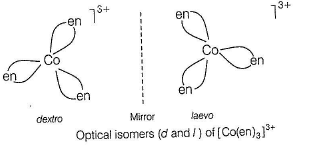Optical isomers have mirror images that cannot be superimposed on one another. These are called as enantiomers. The molecules or ions that cannot be superimposed are called chiral.

QUESTION: 2

### Which of the following types of octahedral complexes will exhibit geometrical isomerism (where, M = metal, a, b = achiral ligands)?

Solution:

Octahedral complexes of formula [Ma4b2] in which the two ligands b may be oriented cis or trans to each other, e.g.

Geometrical isomers (cis and trans) of [Co(NH3)4CI2]+.

QUESTION: 3

### Which of the following can exhibit linkage isomerism?

Solution:

Ligand which can ligate through two different atoms is called ambidentate ligand. NO2- ion can coordinate either through nitrogen or through oxygen to a central metal atom/ion.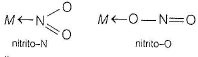Hence,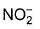ligand show linkage isomerism, e.g. the complex [Co(NH3)5(NO2)]CI2 which is obtained as the red form, in which the nitrite ligand is bound through oxygen (—ONO) and as the yellow form, in which the nitrite ligand is bound through nitrogen (— NO2).

QUESTION: 4

What type of isomerism is shown by [Co(NH3)4Br2]Cl?

Solution:

The complex [Co(NH3)4Br2]CI show geometrical and ionisation isomerism.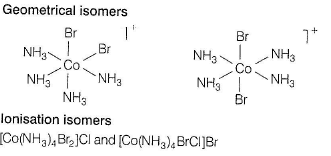QUESTION: 5

Which of the following does not show optical isomerism?

Solution:

Due to symmetry in the complex [Co(NH3)3CI3], it failed to show optical isomerism. The type of coordination entity show fac and mer isomerism or geometrical isomerism.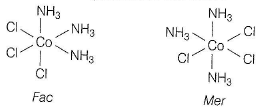The facial (fac) and meridional (mer) isomers of [Co(NH3)3CI3].

QUESTION: 6

The ionisation isomer of [Cr(H2O)4 CI(NO2)]CI is

Solution:

Ionisation isomerism is due to the exchange of groups between the complex ion and the ions outside it, e.g. [Cr(H2O)4CI(NO2)CI] and [Cr(H2O)4CI2]NO2 show ionisation isomerism.

QUESTION: 7

Which of the following complex species is not expected to exhibit optical isomerism?

Solution:

[CoNH3CI3] do not exhibit optical isomerism. Optical isomerism is shown by M(AA)2X2 type, M(AA)3 type , [M(AA) B2X2] type complexes containing hexadentate ligand and MLa Lb Lc Ld Left type.

QUESTION: 8

The complex [Co(NH3)6[Cr(C2O4)3] and [Cr(NH3)6[Co(C2O4)3] exhibit

Solution:

Coordination isomerism is possible when both positive and negative ions of a salt are complex ions and two isomers differ in distribution of ligands in the cation and the anion.

QUESTION: 9

Which of the following exhibit cis-trans isomerism?

Solution:

All the three compounds, i.e. [PtCI2(NH3)2], [PdCl2Brl] and [Pt(NH3)(py)(CI)(Br)] exhibit cis and trans isomerism.

QUESTION: 10

Which of the following is considered to be an anticancer species?

Solution:

cis platin is used as ananticancer drug.

*Multiple options can be correct
QUESTION: 11

Direction (Q. Nos. 11-15) This section contains 5 multiple choice questions. Each question has four choices (a), (b), (c) and (d), out of which ONE or MORE THAN ONE are correct.

Q.

Solution:

Linkage isomerism occurs when two or more atoms in a monodentate ligand may function as a donor, i.e. when an ambidentate ligand is present. e.g.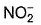, CN- and SCN-.

*Multiple options can be correct
QUESTION: 12

Which is/are correct pair?

Solution:

[CrCI(NH3)5] I2 show ionisation isomerism.
[PtCI4I2] show geometrical isomerism.

*Multiple options can be correct
QUESTION: 13

Coordination isomerism is/are shown by

Solution:

This type of isomerism arises from the interchange of ligands between cationic and anionic entities of different metal ions present in a complex.

*Multiple options can be correct
QUESTION: 14

Which of the following show geometrical isomerism?

Solution:

Due to symmetry in the complex [Cu(NH3)4]CI2, it fails to show geometrical isomerism but all others show.

*Multiple options can be correct
QUESTION: 15

Which of the following statements is/are correct?

Solution:

Geometrical isomerism is not observed in complexes of coordination number 4 having tetrahedral geometry. The platinumglycinato complex [ Pt(gly)2 show geometrical isomerism (cis and trans form).

QUESTION: 16

Comprehension Type

Direction (Q. Nos. 16 and 17) This section contains a paragraph, describing theory, experiments, data etc. Two questions related to the paragraph have been given. Each has only one correct answer among the four given options (a) ,(b), (c) and (d).

Passage

Consider the following isomers of [Co(NH3)4Br2]+. The black sphere represents Co, grey sphere represents NH3 and unshaded sphere represents Br.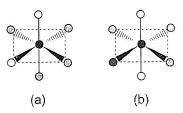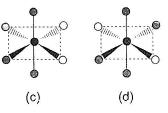Q.

The oxidation state and coordination number of cobalt in the complex [Co(NH3)4Br2]+ are

Solution:

For O.S:

X + 0 + 2(-1) = 1

X = 3

Coordination number is the number of molecules linked to the central atom.

Hence B is the correct answer.

QUESTION: 17

Passage

Consider the following isomers of [Co(NH3)4Br2]+. The black sphere represents Co, grey sphere represents NH3 and unshaded sphere represents Br.Q.

Which of the structures is identical?

Solution:

Structure (a) = structure (c) and, structure (b) = structure (d)

QUESTION: 18

Matching List Type

Direction (Q, Nos. 18 and 19) Choices for the correct combination o f elements from Column I and Column II are given as options (a), (b), (c) and (d) out of which one is correct.

Q.

Match the Column ! with Column II and mark the correct option from the codes given below.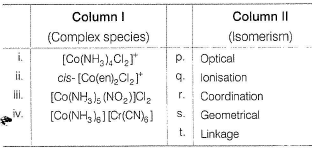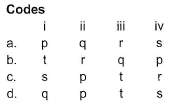Solution:

[Co(NH3)4CI2]+ exhibits geometrical isomerism.
cis-[Co(en)2CI2]+ because of the absence of symmetry elements exhibit optical isomerism.
[Co(NH3)5(NO2)]CI2 because of the presence of ambidentate (NO2) ligand exhibit linkage isomerism. [Co(NH3)6][Cr(CN)6] exhibits coordination isomerism because here, cation and anion both are complex.

(i) → (s), (ii) →(p), (iii) → (t), (iv) → (r)

QUESTION: 19

Match the Column I with Column II and mark the correct option from the codes given below.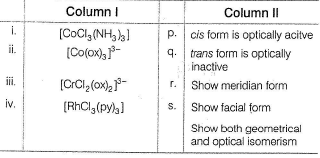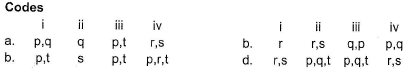Solution:

(i) → (r,s), (ii) → (p,q,t), (iii) → (p,q,t), (iv) → (r,s)

*Answer can only contain numeric values
QUESTION: 20

One Integer Value Correct Type

Direction (Q. Nos. 20-24) This section contains 5 questions. When worked out will result in an integer from 0 to 9 (both inclusive).

Q.

Total number of geometrical isomers for the complex [RhCI(CO)(PPh3)(NH3)] is

Solution:

For a complex of the type MABCD, three geometrical isomers are possible.

*Answer can only contain numeric values
QUESTION: 21

The total number of isomers of [Co(en)2CI2]+ is

Solution:

The complex has cis and trans isomers. The c/s has non-superimposable mirror images. Hence, total are 3.

*Answer can only contain numeric values
QUESTION: 22

The total number of possible isomers for the complex compound [Cull(NH3)4][PtlICI4]

Solution:

[Cu(NH3)3CI][Pt(NH3)CI3], [Cu(NH3)CI3][Pt(NH3)3CI]
[Cu(NH3)4] [PtCI4], [Pt(NH3)4] [CuCI4]

*Answer can only contain numeric values
QUESTION: 23

The number of geometrical isomers possible for Cr(NH3)3CI3 are

Solution:

This is [Ma3b3] type of complex and this has two geometrical isomers called facial (fac isomer) and meridional (mer isomer).

*Answer can only contain numeric values
QUESTION: 24

For square planar complex of platinum (II), [Pt(NH3)(Br)(CI)py]2+, how many isomeric forms are possible?

Solution:

[Mabcd] type complexes exist in three isomeric forms.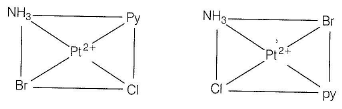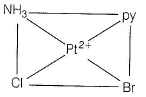QUESTION: 25

Statement Type

Direction ( Q. No. 25) This section is based on Statement I and Statement II. Select the correct answer from the codes given below.

Q.

Statement I : Complexes of MX6 and MX5L type do not show geometrical isomerism. Assume that X and L are unidentate.

Statement Il : Geometrical isomerism is not shown by complexes of coordination number 6.

Solution:

MX6 and MX5L type complexes do not exhibit geometrical isomerism, cis [Co(en)2CI2]+ because of the absence of symmetry elements exhibit optical isomerism.
[Co(NH3)5(NO2)]CI2 because of the presence of ambidentate (NO2) ligand exhibit linkage isomerism. [CoNH3)6][Cr(CN)6] exhibits coordination isomerism because here cation and anion both are complex.